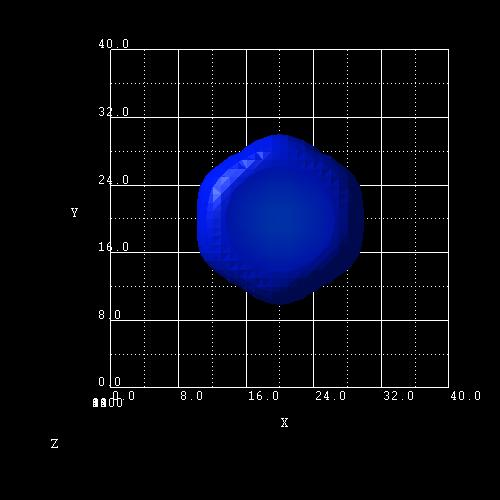nextnano.com  nextnano³  Download | Search | Copyright | Publications  * password protected nextnano³ softwarequantum-regions

# Quantum regions

For quantum solutions, you have to define quantum regions and clusters on which certain quantum models are applied.
The syntax of the quantum regions and clusters is the same as for current and material regions.

For more info on specifiers see ``` \$regions```.```  ```

```!---------------------------------------------------! \$quantum-regions                          optional  !  region-number            integer         required  !  base-geometry            character       required  !  region-priority          integer         required  !                                                     !  x-coordinates            double_array    optional  !  y-coordinates            double_array    optional  !  z-coordinates            double_array    optional  !                                                     !  base-coordinates         double_array    optional  !  top-coordinates          double_array    optional  !                                                     !  corner-coordinates       double_array    optional  !                                                     !  semi-ellipse-base        double_array    optional  !  semi-ellipse-top         double_array    optional  ! \$end_quantum-regions                      optional  ! !---------------------------------------------------!```

## Syntax

```region-number = 1              ! ```an integer number to refer to geometry element```               = 2               = ...  ```

```base-geometry = line           ! ```type of geometry element
```                               ! ```1D:  ```line                                ! ```2D:  ```rectangle ```|` triangle `|``` trapezoid ```|```  polygon ```|``` semiellipse                                ! ```3D:  `cuboid `|``` obelisk ```|` cone `|``` truncated-cone ```|``` semiellipsoid ```|``` hexagonal-obelisk ```|``` triangular-prism```

The figure shows the calculated wave function that was confined by a 3D``` hexagonal-obelisk ```shaped quantum region with Dirichlet boundary conditions.```region-priority = 1                               ! ```a positive integer to set overwriting priority

```x-coordinates = xmin xmax                         ! xmin```,``` xmax ```of` base-geometry` = ```cuboid y-coordinates = ymin ymax                         ! ymin```,``` ymax ``` of` base-geometry` = ```cuboid z-coordinates = zmin zmax                         ! zmin```,``` zmax ``` of` base-geometry` = `cuboid`

```base-coordinates = xmin xmax ymin ymax zmin zmax```

```top-coordinates  = xmin xmax ymin ymax zmin zmax ``` ` ``! `for``` truncated-cone``````                  = x y z ```for semiellipsoid and cone

```corner-coordinates = x1 y1   x2 y2   x3 y3        ! (x1,y1)(x2,y2)(x3,y3)``` triangle corner coordinates, interpreted via orientation

```semi-ellipse-base = imin imax  jmin jmax          ! ```base line ellipse: two pairs of```  xmin,xmax  ymin,ymax  zmin,zmax ``` - one pair equal numbers

```semi-ellipse-top = i j                            ! ```top coordinate pair: e.g. for coordinate orientation `(101) -> (x,z)`